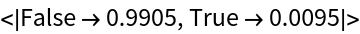#Function Repository Resource:

# ContainsAllMultiplicities

Similar to ContainsAll but taking the multiplicities into consideration

Contributed by: Sander Huisman
 ResourceFunction["ContainsAllMultiplicities"][e1,e2] yields True if e1 contains all of the elements of e2 taking into account multiplicities. ResourceFunction["ContainsAllMultiplicities"][e2] is an operator form that yields True when the object to which it is applied contains all of the elements of e2.

## Examples

### Basic Examples

Check if the elements of the second list are all contained in the first list:

 In:=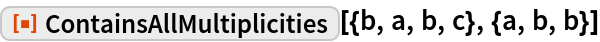Out=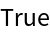Check if the elements of the second list are all contained in the first list:

 In:=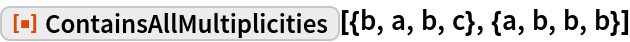Out=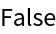### Scope

Make a function that checks if a list has one 1, two 2s and one 3:

 In:=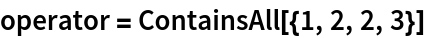Out=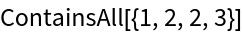Apply to a bunch of lists:

 In:=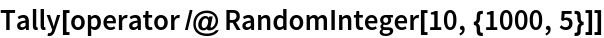Out=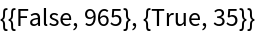### Properties and Relations

Elements of e1 and e2 can be lists themselves:

 In:=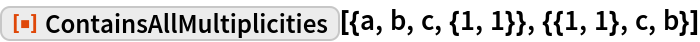Out=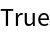There are not enough c’s in the first list:

 In:=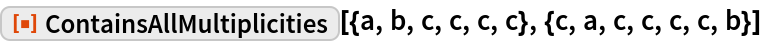Out=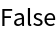Check the same but without considering the multiplicities:

 In:=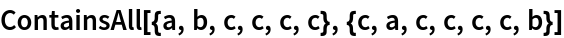Out=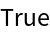If e1 is empty and e2 is non-empty, the answer is always False:

 In:=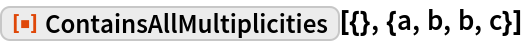Out=If e2 is empty, the answer is always True:

 In:=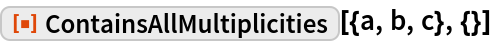Out=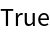If e1 and e2 are empty, the answer is True:

 In:=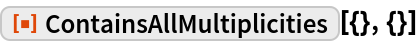Out=### Neat Examples

What are the chances that two randomly generated lists of length 10 overlap exactly:

 In:=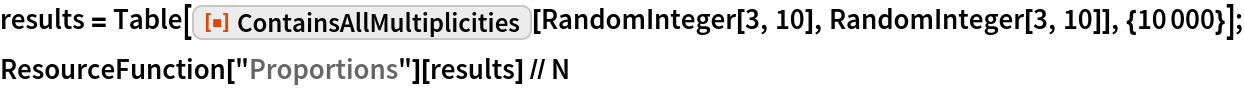Out=